## Quadratic Equations of Class 10

A polynomial of degree 2 (i.e.ax2 + bx + c) is called a quadratic polynomial where a ≠ 0 and a, b, c are real numbers.

### GENERAL FORM OF A QUADRATIC EQUATION:

The general form of quadratic equation is ax2 + bx + c = 0, where a,b,c are real numbers and a ≠ 0 Since a ≠ 0, quadratic equations, in general are of the following types :-

(i) b = 0, c ≠ 0i.e., of the type ax2 + c = 0.

(ii) b ≠ 0c = 0, i.e. of the type ax2 + bx = 0.

(iii) b = 0, c = 0, i.e. of the type ax2 = 0.

(iv) b ≠ 0, c ≠ 0i.e., of the type ax2 + bx + c = 0.

### CLASSIFICATION OF A QUADRATIC EQUATION:

It is classified into two categories:

(i) Pure quadratic equation (of the form ax2 + c = 0 i.e., b = 0  in ax2 + bx + c)

e.g. x2 - 4 = 0 and 3x2 + 1 = 0 are pure quadratic equations.

(ii) Affected quadratic equation (of the form ax2 + bx + c = 0, b ≠ 0).

e.g. x2- 2x - 8 = 0 and 5x2 + 3x - 2 = 0 are affected quadratic equations.

For a quadratic polynomial p(x) = ax2 + bx + c, those values of x for which ax2 + bx + c = 0 is satisfied, are called zeros of quadratic polynomial p(x), i.e. if p(α) = aα2 + bα + c = 0 then α is called the zero of quadratic polynomial.

### ROOTS OF A QUADRATIC EQUATION:

The value of x which satisfies the given quadratic equation is known as its root. The roots of the given equation are known as its solution.

General form of a quadratic equation is :

ax2 + bx + c = 0

or 4a2x2 + 4abx + 4ac = 0 [Multiplying by 4a]

or 4a2x2 + 4abx = –4ac [By adding b2 both sides]

or 4a2x2 + 4abc + b2 = b2 – 4ac

or (2ax + b)2 = b2 – 4ac

Taking square root of both the sides

2ax + b =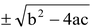or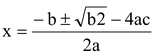Hence, roots of the quadratic equation ax2 + bx + c = 0 are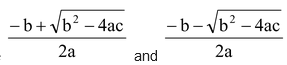### REMARK:

A quadratic equation is satisfied by exactly two values of ‘a’ which may be real or imaginary. The equation, ax2 + bx + c = 0 is :

• A quadratic equation if a ≠ 0 Two roots
• A linear equation if a = 0, b ≠ 0 One root
• A contradiction if a = b = 0, c ≠ 0 No root
• An identify if a = b = c = 0 Infinite roots
• A quadratic equation cannot have more than two roots.
• If follows from the above statement that if a quadratic equation is satisfied by more than two values of x, then it is satisfied by every value of x and so it is an identity.

### NATURE OF ROOTS :

Consider the quadratic equation, ax2 + bx + c = 0 having αβ as its roots and b2 – 4ac is called discriminate of roots of quadratic equation. It is denoted by D or Δ.

Roots of the given quadratic equation may be

(i) Real and unequal (ii) Real and equal (iii) Imaginary and unequal.

Let the roots of the quadratic equation ax2 + bx + c = 0 (where a ≠ 0,b,c ∈ R ) be α  and β then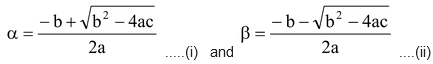The nature of roots depends upon the value of expression ‘b2 - 4ac’ within the square root sign. This is known as discriminate of the given quadratic equation.

Consider the Following Cases:

Case-1 When b2 - 4ac > 0, (D > 0)

In this case roots of the given equation are real and distinct and are as follows(i) When a ≠ 0,b,c ∈ Q  and b2 - 4ac is a perfect square

In this case both the roots are rational and distinct.

(ii) When a ≠ 0,b,c ∈ Q and b2 - 4ac is not a perfect square

In this case both the roots are irrational and distinct. [See remarks also]

Case-2 When b2 - 4ac = 0, (D = 0)

In this case both the roots are real and equal to -b/2a.

Case-3 When b2 - 4ac < 0, (D < 0)

In this case b2 – 4ac < 0, then 4ac – b2 > 0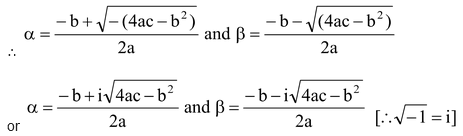i.e. in this case both the root are imaginary and distinct.

### REMARKS:

• If a,b,c ∈ Q and b2 - 4ac is positive (D > 0) but not a perfect square, then the roots are irrational and they always occur in conjugate pairs like 2 + √3 and 2- √3. However, if a, b, c are irrational number and b2 – 4ac is positive but not a perfect square, then the roots may not occur in conjugate pairs.
• If  2 – 4ac is negative (D > 0), then the roots are complex conjugate of each other. In fact, complex roots of an equation with real coefficients always occur in conjugate pairs like 2 + 3i and 2 – 3i. However, this may not be true in case of equations with complex coefficients. For example, x2 – 2ix – 1 = 0 has both roots equal to i.
•  If a and c are of the same sign and b has a sign opposite to that of a as well as c, then both the roots are positive, the sum as well as the product of roots is positive (D ≥ 0).
•  If a,b, are of the same sign then both the roots are negative, the sum of the roots is negative but the product of roots is positive (D ≥ 0).

question 1. Check whether the following equations are quadratic or not.

(i)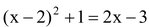(ii)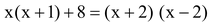(iii)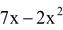(iv)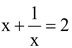Solution: (i)can be rewritten as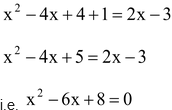It is of the form ax2 + bx + c = 0

Therefore, the given equation is a quadratic equation.

(ii) Asand (x + 2) (x - 2) = x2 - 4

Therefore, we have x2  + x + 8 = x2 - 4

⇒ x + 12 = 0

It is not of the form ax2 + bx + c = 0

Therefore, the given equation is not a quadratic equation.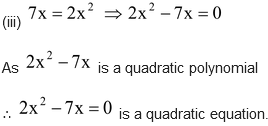(iv)⇒ x + x-1 - 2 = 0

Since x + x-1 - 2 is not a quadratic polynomial.is not a quadratic equation

question 2. In each of the following, determine the value of k for which the given value is a solution of the equation.

(i)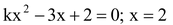(ii)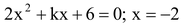Solution: (i)…(i)

Since x = 2 is a solution of the equation

Now substituting x = 2 in equation (i), we get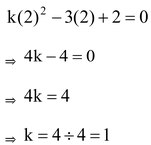(ii) Since x = –2 is a solution of the equation…(i)

Now substituting x = – 2 in (i), we get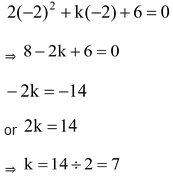Do solve NCERT text book for class 10 maths with the help of pw NCERT solutions for class 10 maths.